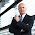## xts object - subscript out of bounds

I bet you have seen this error a few times. When I compare large xts objects with different number of observations it would hit me right towards the end of the analysis.
I wrote a small function, which allows me to check the length of the sets in advance and retains only the symbols, which hold the exact number of observations required.
benchmark is simply my ticker with the number of observations I would like to see.

checkrow <- function(Tickers,Bench,From,To)
{
getSymbols(Bench, index.class="POSIXct", from = From, to = To,src="yahoo")
my = NA
y=get(benchmark)
m=NROW(y)
for(sticker in tickers){

x=get(sticker)

if( NROW(x)<m)
{
removeSymbols(sticker)
}
if( NROW(x)==m)
{
a=NROW(my)
my[a+1]=sticker

}

}
my = my[-1]
tickers = my
return(tickers)
}

#### 1 comment :

1.what you could do as well is use the na.locf function and replace NA with the next closest observation.

frame = get(benchmark)
for(symbol in tickers){
x=get(symbol)
s=cbind(x,frame)
s=na.locf(s,na.rm=T)
x=s[,1:6]
assign(symbol,x)
}